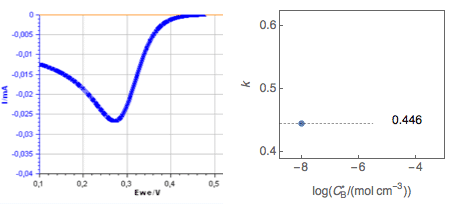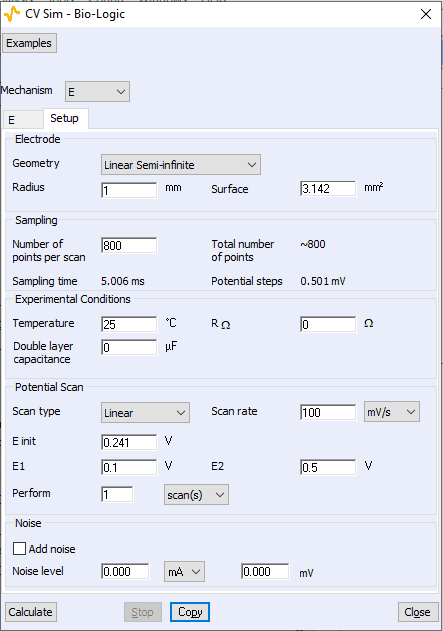Topic 10 min read# CVSim as a learning tool. I. Diffusion coefficient

Latest updated: March 25, 2022

CVSim is the powerful cyclic voltammetry simulation tool available in EC-Lab®, the control and analysis software for BioLogic potentiostats. CVSim is a great educational tool to help understand how cyclic voltammetry curves can be used to access the kinetic parameters of redox reactions. These simulations allow us to understand the use of cyclic voltammetry without even using a potentiostat!

This topic describes an example.

For a reversible or Nernstian redox reaction (E):

$$\text A + z \text e \leftrightarrow \text B$$

The peak current $I_\text p$ studied by cyclic voltammetry varies linearly with the square root of the potential scan rate $v$ with [1-3]:

$$I_\text p=-k\,A\, z \,F\,C_\text A\sqrt{z\, f_\text N\,v\,D_\text A} \Rightarrow k=- I_\text p /( A\, z \,F\,C_\text A\sqrt{z\, f_\text N\,v\,D_\text A})$$

and $k=0.446$, $f_\text N=F/(R\,T)$.

As shown in , this relationship can be used to determine the diffusion coefficient of the reducing species $\text A$.

However, it must be pointed out that this relationship is only valid when B is initially absent from the solution, i.e. $C_\text B=0$.

If this is not the case, if $C_\text B\ne 0$, the values of the constant $k$ will change.

These values can be determined using CV Sim , a tool designed for the simulation of cyclic voltammetry curves resulting from a voltammetry experiment, with

$$E(t) = E_\text{init}-v\,t$$

Where the initial potential $E_\text{init}$ is given by:

$$E_\text{init}=E^\text o +\frac{1}{z\, f_\text N} \ln \frac{C_\text A}{C_\text B}$$

As an example, the change of voltammograms for a reversible redox reaction is shown in Fig.1, as well as the change in the value of the $k$ parameter with the $C_\text B$ concentration.Figure 1: Change of voltammogram for a Nernstian redox system with increasing $C_\text B$ concentration

for $D_\text A = D_\text B= 10^{-6}\, \text{cm}^2\, \text{s}^{-1}$, $k^\text o=100\,\text{cm s}^{-1}$, $v=0.1\, \text V \, \text s^{-1}$.Figure 2: Setup conditions used to simulate the curves shown In Fig. 1

 A. J. Bard and L. R. Faulkner. Electrochemical methods. Fundamentals and Applications. John Wiley & Sons, New York, 1980.

 J.-P. Diard, B. Le Gorrec and C. Montella, Cinétique électrochimique, Hermann, Paris, 1996.

 Application note #41-1. CV Sim Simulation of the simple redox reaction (E) Part I. The effect of the scan rate. https://www.biologic.net/documents/cv-simulation-1-electrochemistry-application-note-41-1/

CV Sim Cyclic voltammetry Redox reaction (E) Ec-Lab Potentiostat Potentiostat / Galvanostat